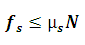# Static Friction Calculator

This Calctown Calculator calculates the maximum static friction that will be offered before relative motion begins between the body and the surface.

N

#### Result

Nwhere

fs = static friction

μs = coefficient of static friction

N = normal force acting perpendicular to point of contact between the body and the surface